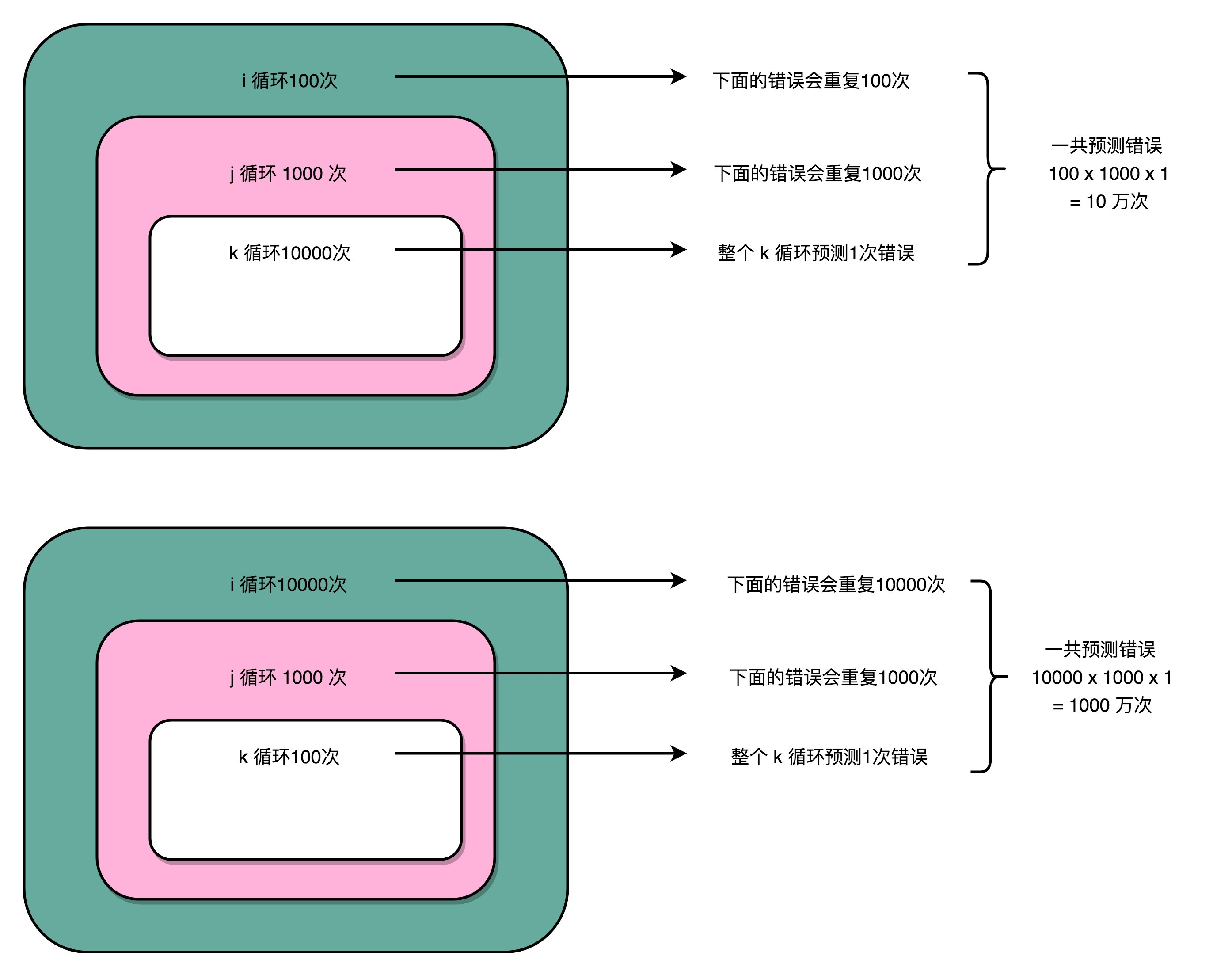25 冒险和预测（四）：今天下雨了，明天还会下雨么？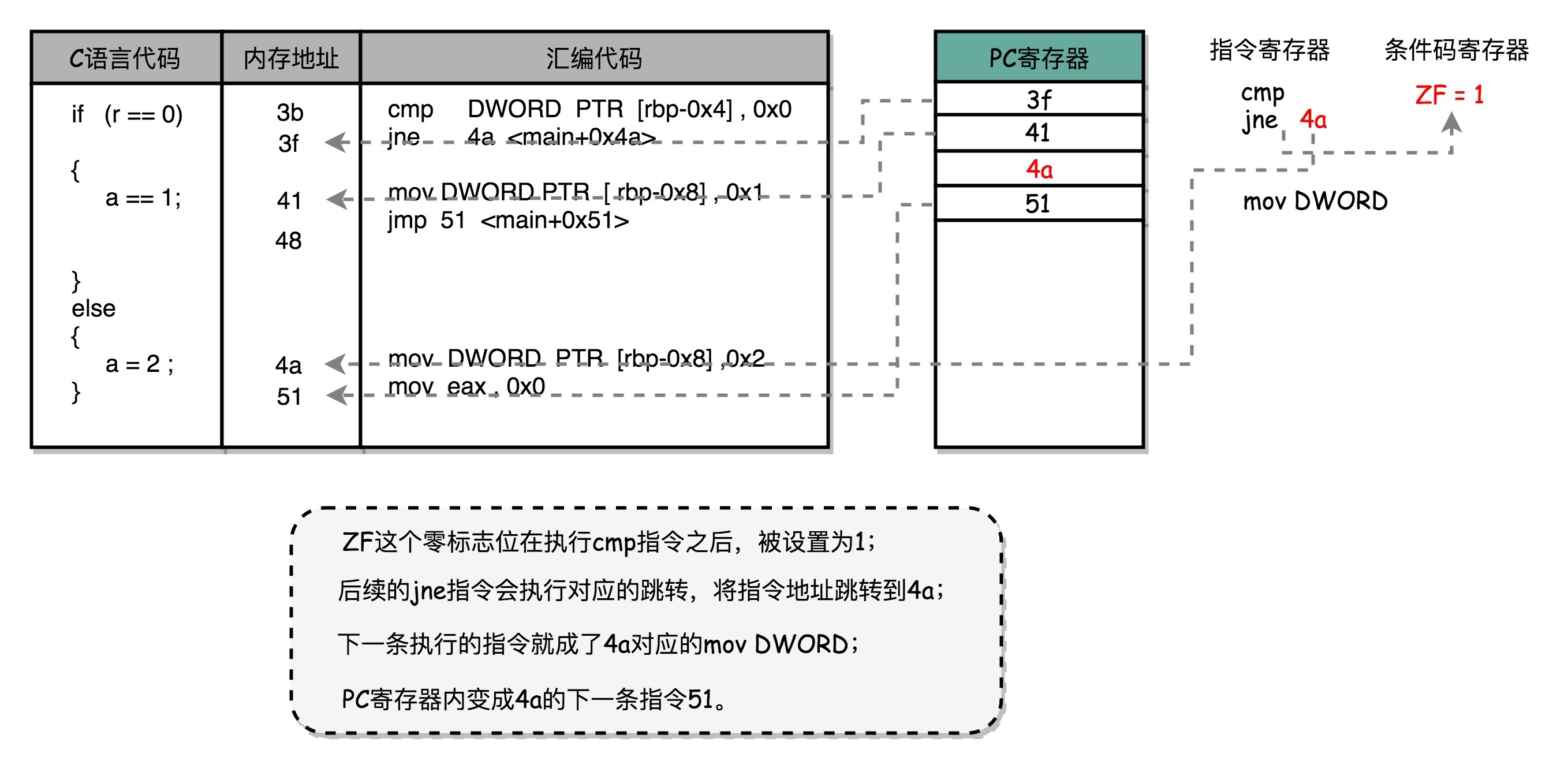分支预测：今天下雨了，明天还会继续下雨么？

分支预测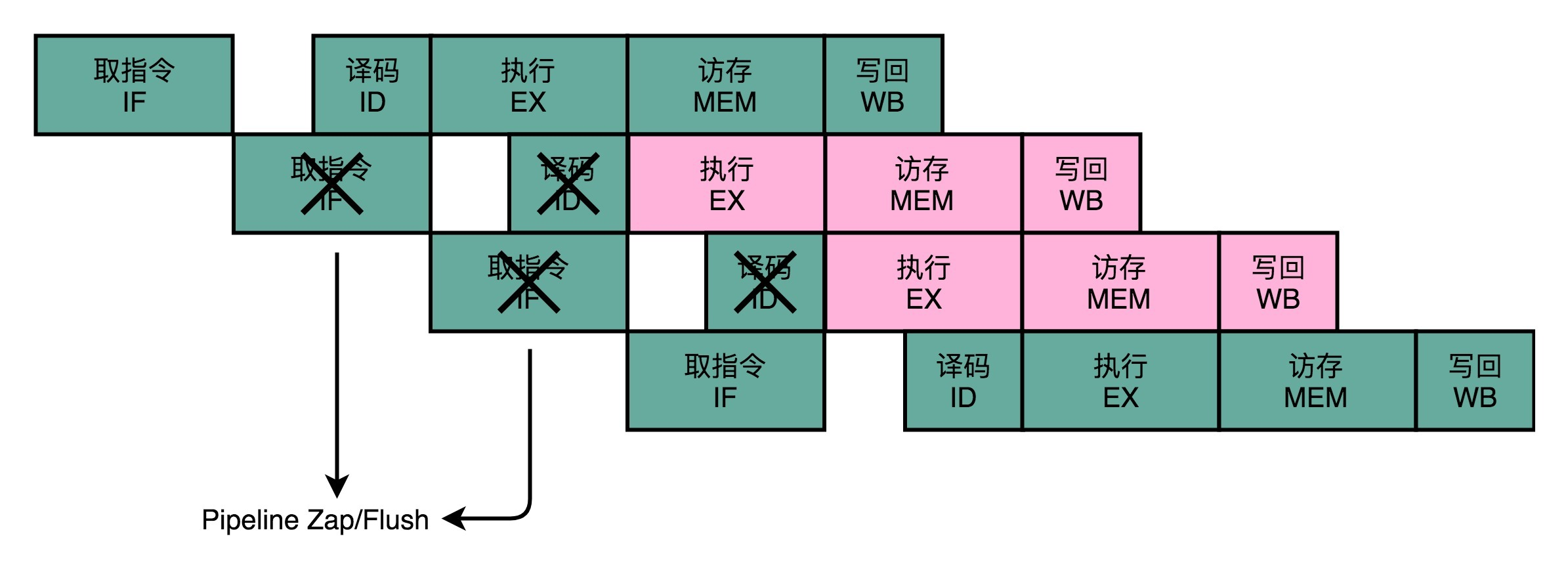动态分支预测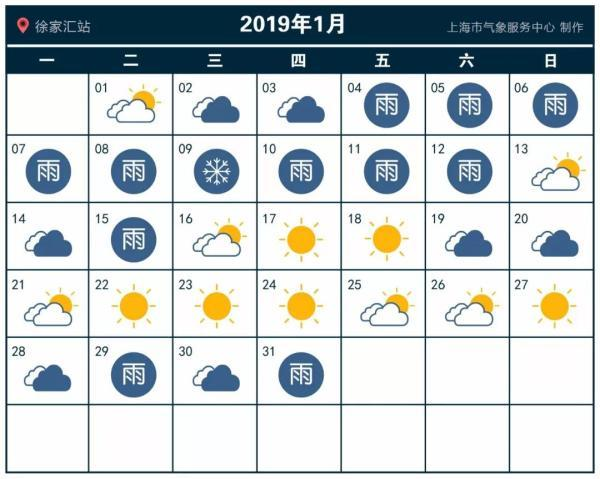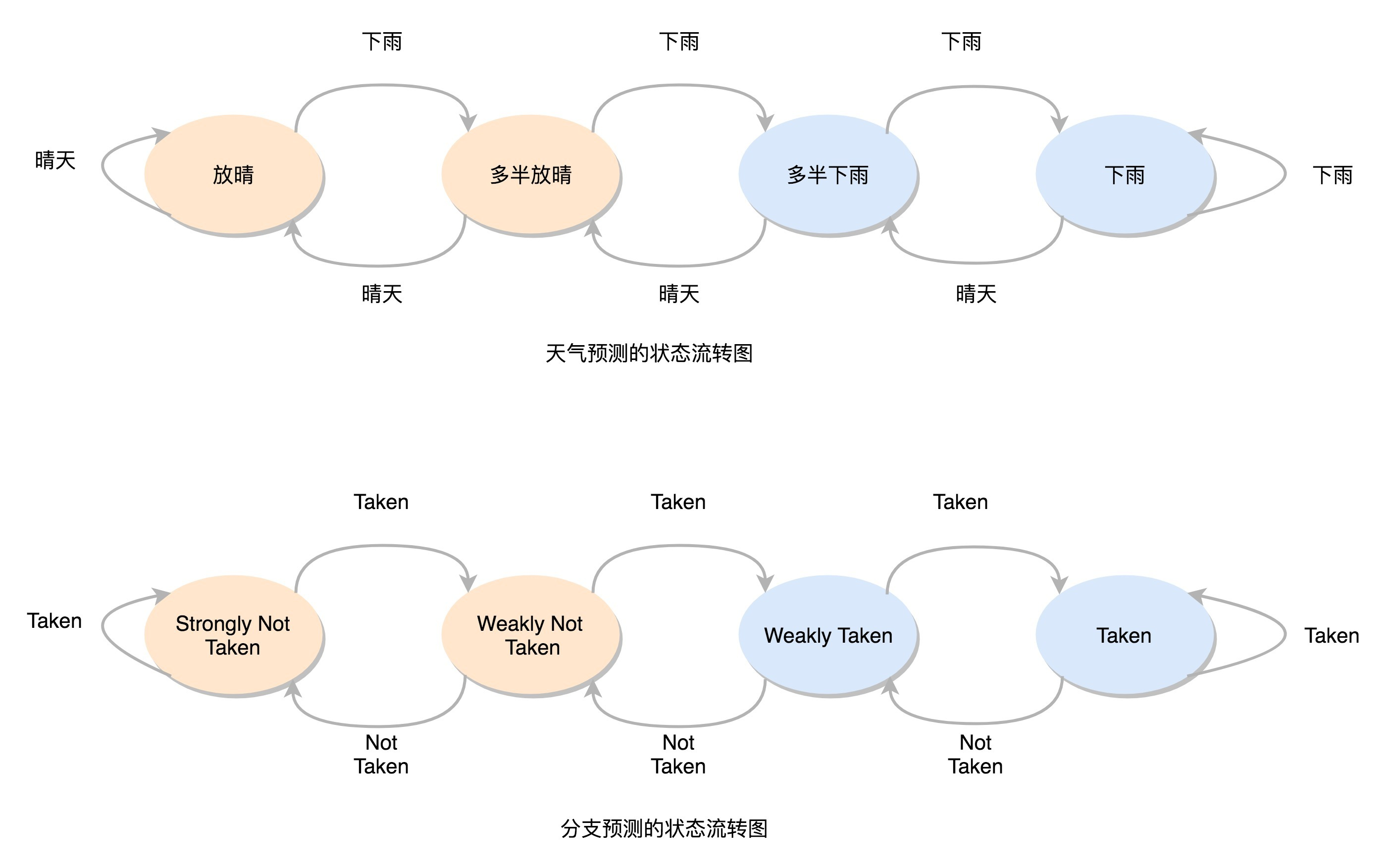为什么循环嵌套的改变会影响性能？

public class BranchPrediction {
public static void main(String args[]) {
long start = System.currentTimeMillis();
for (int i = 0; i < 100; i++) {
for (int j = 0; j <1000; j ++) {
for (int k = 0; k < 10000; k++) {
}
}
}
long end = System.currentTimeMillis();
System.out.println("Time spent is " + (end - start));

start = System.currentTimeMillis();
for (int i = 0; i < 10000; i++) {
for (int j = 0; j <1000; j ++) {
for (int k = 0; k < 100; k++) {
}
}
}
end = System.currentTimeMillis();
System.out.println("Time spent is " + (end - start) + "ms");
}
}

Time spent in first loop is 5ms
Time spent in second loop is 15ms